Name:    Practice Final B

Multiple Choice
Identify the choice that best completes the statement or answers the question.

1.

For bromine,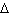H° vap = 30.91 kJ/mol andS° vap = 93.23 J/Kmol.   What is the normal boiling point for bromine?
 A) 332°C B) 124°C C) 25°C D) 58°C

2.

The formation constant (Kf) for the reaction shown below is 1.7x107 at 25 °C.  What isG° at this temperature?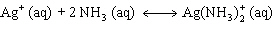A) –41.2 kJ B) –17.9 kJ C) –1.5 kJ D) –3.5 kJ

3.

For a particular processG is less thanH.  Therefore
 A)S is zero B)S is positive C)S is negative D)S is positive ifH is negative andS is negative ifH is positive

4.

Elemental boron can be formed by the reaction of boron trichloride and hydrogen, as shown below.  IfS° for this reaction is 80.3 J/K, what is S° for BCl3(g)?

 BCl3(g)        +       1.5 H2(g)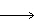B(s)         +   3 HCl(g) S° (J/Kmol) ??? 130.6 5.87 186.8
 A) 18.2 J/Kmol B) 290.1 J/Kmol C) 450.6 J/Kmol D) –18.2 J/Kmol

5.

Doubling all the coefficients in the equation for a cell reaction
 A) does not change E° orG° B) doubles both E° andG° C) doubles E°, but does not changeG° D) doublesG°, but does not change E°

6.

CalculateG° for a cell that utilizes oxidation of copper by nitric acid at 25 °C.

Cu2+(aq) + 2 eCu(s)                              E° = 0.34 V
NO3(aq) + 4 H+(aq) + 3 eNO(g) + 2 H2O(l)         E° = 0.96 V
 A) –360 kJ B) –120 kJ C) –240 kJ D) –180 kJ

7.

Which of the following substances is the strongest oxidizing agent?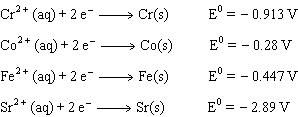A) Cr2+ B) Co2+ C) Fe2+ D) Sr2+

8.

Predict the sign ofS° for the following reaction:

K2SO4 (s)2 K+ (aq) + SO42– (aq)
 A)S° > 0 B)S° = 0 C)S° < 0 D) More information is needed to make a reasonable prediction

9.

Which of the following substances will reduce Ag+ but not Cu2+?
 A) Br2 B) Sn2+ C) Fe3+ D) I2

10.

Which of the following substances is the strongest reducing agent?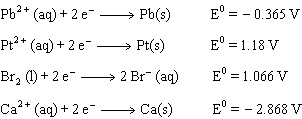A) Pb(s) B) Pt(s) C) Br–(aq) D) Ca(s)

11.

In an electrochemical cell, Q= 0.0010 and K=0.10.  Which statement below is true?
 A) Ecell is negative and E°cell is positive B) Ecell is positive and E°cell is negative C) Both Ecell and E°cell are positive D) Both Ecell and E°cell are negative

12.

A redox reaction employed in an elelctrochemical cell has a negative DG°rxnWhich statement is true?
 A) E°cell is positive; K <1 B) E°cell is negative; K <1 C) E°cell is positive; K >1 D) E°cell is negative; K >1